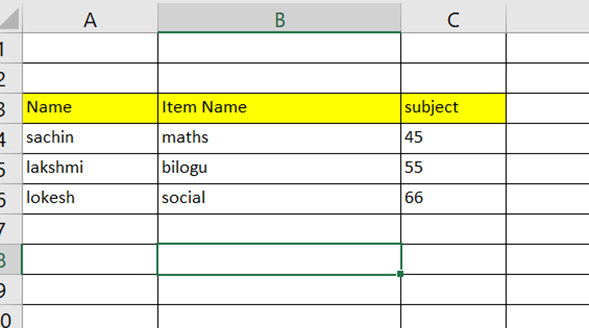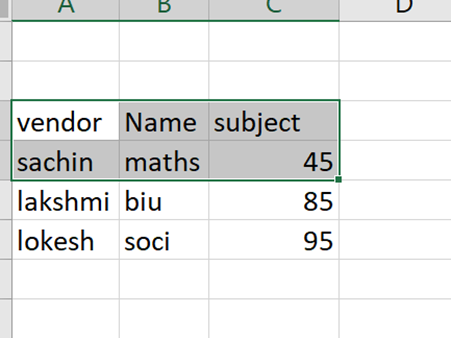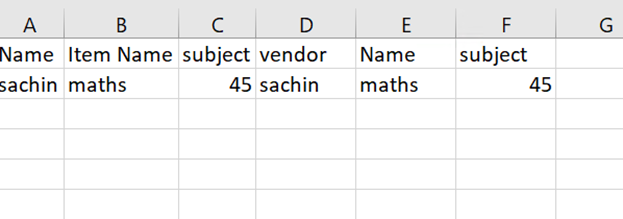# Compare two different excel columns and matched remove data in second excel sheet

Hi,

I have two excel file,

One excel, there is an column with " Itemname"
Another excel has column " Name"

Now i need to coampare two two columns and remove the matched data in second sheet

Use the below linq queries

Datatable1.AsEnumerable.Where(Function(x) Not Datatable2.AsEnumerable.Any(Function(y) y(“Itemname”).toString = x(“Name”).toString)).CopyToDataTable

Hope it helps!!

Can you try with this LINQ expression

`````` DT_2.AsEnumerable.Where(Function(r) not DT_1.AsEnumerable.Any(Function(r2) r2("Itemname").ToString=r("Name").ToString)).CopyToDataTable.DefaultView.ToTable(False,{"Column1","Column2","Column3"})
``````

Regards
Gokul

firstsheet.xlsx (8.9 KB)
secondsheet.xlsx (120.0 KB)

1. Read the data from both Excel files into separate DataTable variables:
• Use the “Read Range” activity to read the data from the first Excel file into a DataTable variable. Let’s name it `dtFirstFile`.
• Use the “Read Range” activity to read the data from the second Excel file into another DataTable variable. Let’s name it `dtSecondFile`.
1. Identify the common column names in both DataTables:
• Determine the column name in `dtFirstFile` that corresponds to “Itemname” and the column name in `dtSecondFile` that corresponds to “Name”. Note down these column names.
1. Remove the matched data from the second DataTable:
• Use the Assign activity to assign the filtered result to a new DataTable variable. Let’s name it `dtFiltered`.
• Set the left-hand side of the Assign activity to `dtFiltered`.
• Set the right-hand side to the following expression:

dtSecondFile.AsEnumerable().Where(Function(row) Not dtFirstFile.AsEnumerable().Any(Function(x) x(“Itemname”).ToString() = row(“Name”).ToString())).CopyToDataTable()

1. Write the filtered data to a new Excel file:
• Use the “Write Range” activity to write the `dtFiltered` DataTable to a new Excel file.
• Specify the desired file path and name for the new Excel file.
• Ensure that you select the appropriate sheet to write the data.

Hope it helps!!

Hi Gokul,

I tried the mentioned query, but error coming, I have shared you the files can you chcek on that please and help me

Hi Pravalika,

There is no common column names,

Just i need to compare the column which i specified.
If matches needs to remove the entire rows in second sheet

Can you please have a look in shared excel

Use the below workflow @Sachin_Sunny

Then use the linq querey below

Datatable1.AsEnumerable.Where(Function(x) not Datatable2.AsEnumerable.Any(Function(y) y(“Name”).toString = x(“Item Name”).toString)).CopyToDataTable

Hope it helps!!

the aboive logic is deleting in first sheet

I want to delete the matched data in second sheet

Make it like this.

Datatable2.AsEnumerable.Where(Function(x) not Datatable1.AsEnumerable.Any(Function(y) y(“Name”).toString = x(“Item Name”).toString)).CopyToDataTable

Take an assign activity in Save to give the Datatable2 in the value give the above linq querey

Can you please send an xaml please. when i use the above emntioned string it is promping as name doesnt find in the table.

Can you send me the xaml it helps for me

Thank you, It worked!!!

1 Like

Than you @Sachin_Sunny. If you get the solution for your query. Make Mark as Solution to close the loop.

Hi sir,

One small question now we did delete the rows in second excel right.

Now i need to compare both the column and put the result which are equal in new excel.

Can you send me the linq query please?

Take an assign activity create a new Datatable variable like Datatable3. In the value give the below one.
Datatable1.AsEnumerable.Where(Function(x) Datatable2.AsEnumerable.Any(Function(y) y(“Candidates”).toString = x(“Names”).toString)).CopyToDataTable

Hope it helps!!

hi,

I have on e excel as follows:I have another excel sheet as follows:if item name= name then the output should be as follows: